# B.5 Imaginary Time Operators

At finite temperature under thermodynamic equilibrium the state of a system is described by the equilibrium density operator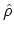. For a giventhe ensemble average of any operator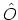can be calculated as (see (3.11))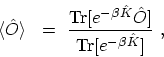(B.25)

where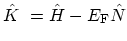may be interpreted as a grand canonical HAMILTONian. For any SCHRÖDINGER operator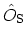, the so called modified HEISENBERG and interaction pictures can be introduced as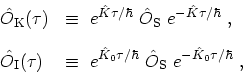(B.26)

where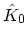includes only the non-interacting part of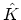. It should be noticed that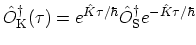is not the adjoint of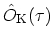as long as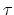is real. Ifis interpreted as a complex variable, however, it may be analytically continued to a pure imaginary value. The resulting expression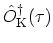then becomes the true adjoint ofand is formally identical with the original HEISENBERG picture defined in (B.12), apart from the substitution offor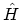. For this reason (B.26) are sometimes called imaginary-time operators.

The modified HEISENBERG and interaction pictures are related by (compare (B.13) and (B.14))(B.27)

where the operator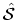is defined by (compare (B.17))(B.28)

Note thatis not unitary, but it still satisfies the group property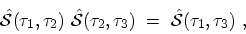(B.29)

and the boundary condition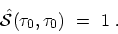(B.30)

In addition, the equation of motion ofis calculated as(B.31)

where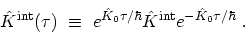(B.32)

It follows that the operatorobeys essentially the same differential equation as the unitary operator introduced in (B.15), and one may immediately write down the solution (compare (B.24))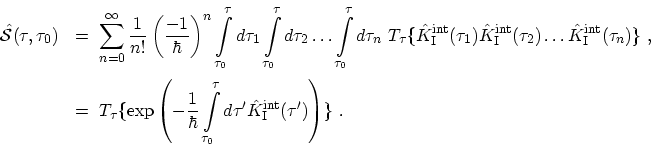(B.33)

Ifis set equal to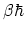, (B.28) may be rewritten as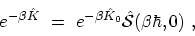(B.34)

which relates the many particle density operator to the single-particle density operator by means of an imaginary time-evolution operator. M. Pourfath: Numerical Study of Quantum Transport in Carbon Nanotube-Based Transistors